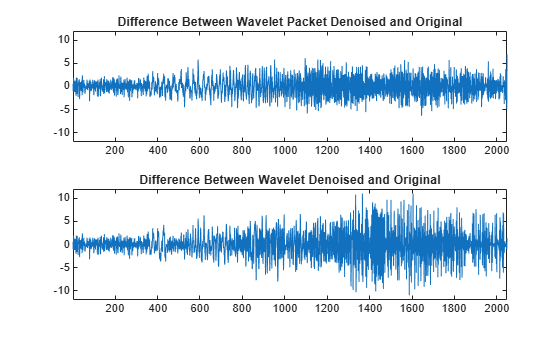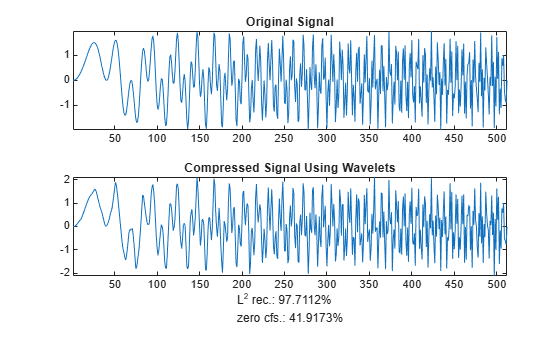wpdencmp

Denoising or compression using wavelet packets

Description

wpdencmp performs a denoising or compression process of a signal or image using wavelet packets. The ideas and procedures for denoising and compression using either wavelet or wavelet packet decompositions are the same. See wdenoise or wdencmp for more information.

example

[xd,treed,perf0,perfl2] = wpdencmp(x,sorh,n,wname,crit,par,keepapp) returns a denoised or compressed version xd of the input data x obtained by wavelet packet coefficient thresholding. wpdencmp also returns the wavelet packet best tree decomposition treed of xd (see besttree for more information), and the L2 energy recovery and compression scores in percentages as perfl2 and perf0, respectively.

[___] = wpdencmp(tree,sorh,crit,par,keepapp) uses the wavelet packet decomposition tree of the data to be denoised or compressed.

Examples

collapse all

This example shows how to denoise using wavelet packets.

Use wnoise to generate the heavy sine signal and a noisy version.

init = 1000;
[xref,x] = wnoise(5,11,7,init);
figure
subplot(2,1,1)
plot(xref)
axis tight
title('Heavy Sine')
subplot(2,1,2)
plot(x)
axis tight
title('Noisy Heavy Sine')Denoise the noisy signal using a four-level wavelet packet decomposition. Use the order 4 Daubechies least asymmetric wavelet.

n = length(x);
thr = sqrt(2*log(n*log(n)/log(2)));
xwpd = wpdencmp(x,'s',4,'sym4','sure',thr,1);

Compare with a wavelet-based denoising result. Use wdenoise with comparable input arguments. Plot the differences between the two denoised signals and original signal.

xwd = wdenoise(x,4,'Wavelet','sym4','DenoisingMethod','UniversalThreshold','ThresholdRule','Hard');
figure
subplot(2,1,1)
plot(x-xwpd)
axis tight
ylim([-12 12])
title('Difference Between Wavelet Packet Denoised and Original')
subplot(2,1,2)
plot(x-xwd)
axis tight
ylim([-12 12])
title('Difference Between Wavelet Denoised and Original')This example shows how to denoise an image using wavelet packets.

Load an image and generate a noisy copy. For reproducibility set the random seed.

rng default
x = X/18 + randn(size(X));
imagesc(X)
colormap(gray)
title('Original Image')figure
imagesc(x)
colormap(gray)
title('Noisy Image')Denoise the noisy image using wavelet packet decomposition. Use ddencmp to determine denoising parameters. Do a three-level decomposition with the order 4 Daubechies least asymmetric wavelet.

[thr,sorh,keepapp,crit] = ddencmp('den','wp',x);
xd = wpdencmp(x,sorh,3,'sym4',crit,thr,keepapp);
figure
imagesc(xd)
colormap(gray)
title('Denoised Image')This example shows how to compress a 1-D signal using wavelet packets.

Load a signal. Use ddencmp to determine compression values for that signal.

x = sumlichr;
[thr,sorh,keepapp,crit] = ddencmp('cmp','wp',x)
thr = 0.5193
sorh =
'h'
keepapp = 1
crit =
'threshold'

Compress the signal using global thresholding with threshold best basis. Use the order 4 Daubechies least asymmetric wavelet and do a three-level wavelet packet decomposition.

[xc,wpt,perf0,perfl2] = wpdencmp(x,sorh,3,'sym4',crit,thr,keepapp);

Compare the original signal with the compressed version.

subplot(2,1,1)
plot(x)
title('Original Signal')
axis tight
subplot(2,1,2)
plot(xc)
xlabel(['L^2 rec.: ',num2str(perfl2),'%   zero cfs.: ',num2str(perf0),'%'])
title('Compressed Signal Using Wavelet Packets')
axis tightCompress the signal again, but this do a three-level wavelet decomposition. Keep all the other parameters the same.

[thr,sorh,keepapp] = ddencmp('cmp','wv',x);
[xcwv,~,~,perf0wv,perfl2wv] = wdencmp('gbl',x,'sym4',3,thr,sorh,keepapp);
figure
subplot(2,1,1)
plot(x)
title('Original Signal')
axis tight
subplot(2,1,2)
plot(xc)
xlabel(['L^2 rec.: ',num2str(perfl2wv),'%   zero cfs.: ',num2str(perf0wv),'%'])
title('Compressed Signal Using Wavelets')
axis tightA larger fraction of coefficients are set equal to 0 when compressing using a wavelet packet decomposition.

Input Arguments

collapse all

Input data to denoise or compress, specified by a real-valued vector or matrix.

Data Types: double

Wavelet packet decomposition of the data to be denoised or compressed, specified as a wavelet packet tree. See wpdec and wpdec2 for more information.

Type of thresholding to perform:

• 's' — Soft thresholding

• 'h' — Hard thresholding

Wavelet packet decomposition level, specified as a positive integer.

Name of wavelet, specified as a character vector or string scalar, to use for denoising. See wavemngr for more information.

Entropy type, specified as one of the following:

Entropy Type (crit)

Threshold Parameter (par)

'shannon'

par is not used.

'log energy'

par is not used.

'threshold'0 ≤ par

par is the threshold.

'sure'0 ≤ par

par is the threshold.

'norm'1 ≤ par

par is the power.

'user'Character vector

par is a character vector containing the file name of your own entropy function, with a single input x.

'FunName'No constraints on par

FunName is any character vector other than the previous entropy types listed.

FunName contains the file name of your own entropy function, with x as input and par as an additional parameter to your entropy function.

crit and threshold parameter par together define the entropy criterion used to determine the best decomposition. See wentropy for more information.

If crit = 'nobest', no optimization is done, and the current decomposition is thresholded.

Threshold parameter, specified by a real number, character vector, or string scalar. par and the entropy type crit together define the entropy criterion used to determine the best decomposition. See wentropy for more information.

Data Types: double

Threshold approximation setting, specified as either 0 or 1. If keepapp = 1, the approximation coefficients cannot be thresholded. If keepapp = 0, the approximation coefficients can be thresholded.

Data Types: double

Output Arguments

collapse all

Denoised or compressed data, returned as a real-valued vector or matrix. xd and x have the same dimensions.

Wavelet packet best tree decomposition of xd, returned as a wavelet packet tree.

Compression score, returned as a real number. perf0 is the percentage of thresholded coefficients that are equal to 0.

L2 energy recovery, returned as a real number. perfl2 is equal to If x is a one-dimensional signal and wname an orthogonal wavelet, perfl2 simplifies to $\frac{100{‖xd‖}^{2}}{{‖x‖}^{2}}.$

 Antoniadis, A., and G. Oppenheim, eds. Wavelets and Statistics. Lecture Notes in Statistics. New York: Springer Verlag, 1995.

 Coifman, R. R., and M. V. Wickerhauser. “Entropy-Based Algorithms for Best Basis Selection.” IEEE Transactions on Information Theory. Vol. 38, Number 2, 1992, pp. 713–718.

 DeVore, R. A., B. Jawerth, and B. J. Lucier. “Image Compression Through Wavelet Transform Coding.” IEEE Transactions on Information Theory. Vol. 38, Number 2, 1992, pp. 719–746.

 Donoho, D. L. “Progress in Wavelet Analysis and WVD: A Ten Minute Tour.” Progress in Wavelet Analysis and Applications (Y. Meyer, and S. Roques, eds.). Gif-sur-Yvette: Editions Frontières, 1993.

 Donoho, D. L., and I. M. Johnstone. “Ideal Spatial Adaptation by Wavelet Shrinkage.” Biometrika. Vol. 81, 1994, pp. 425–455.

 Donoho, D. L., I. M. Johnstone, G. Kerkyacharian, and D. Picard. “Wavelet Shrinkage: Asymptopia?” Journal of the Royal Statistical Society, series B. Vol. 57, Number 2, 1995, pp. 301–369.

Wavelet Toolbox DocumentationGet trial now